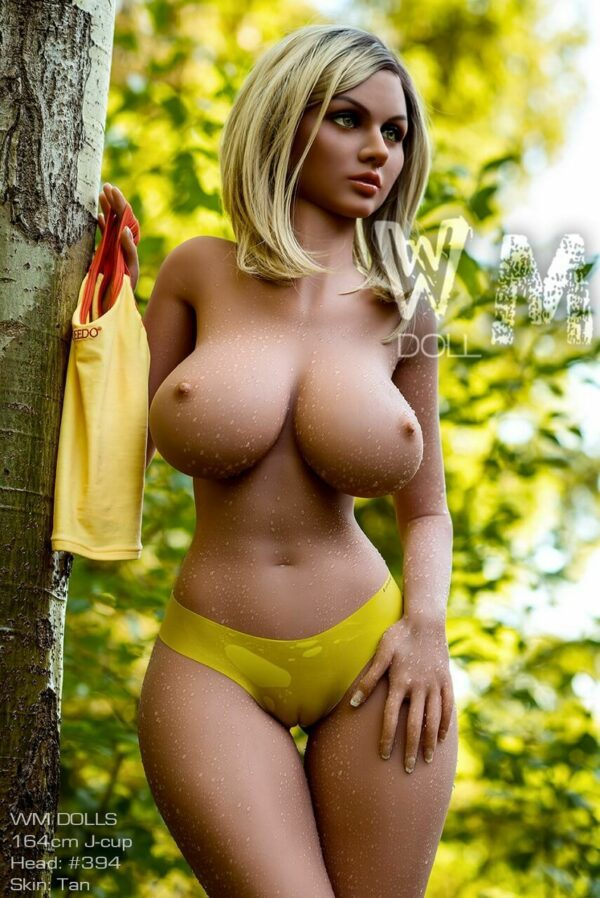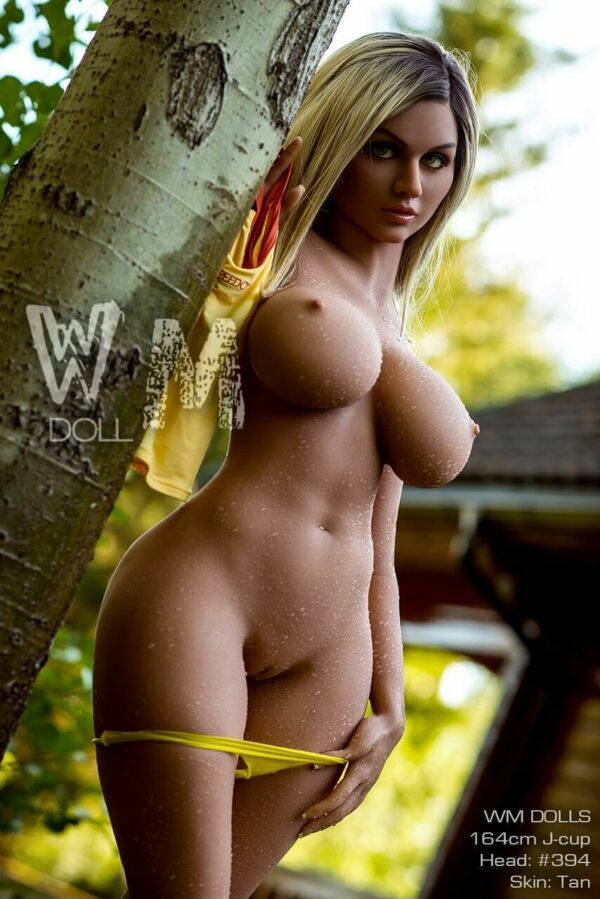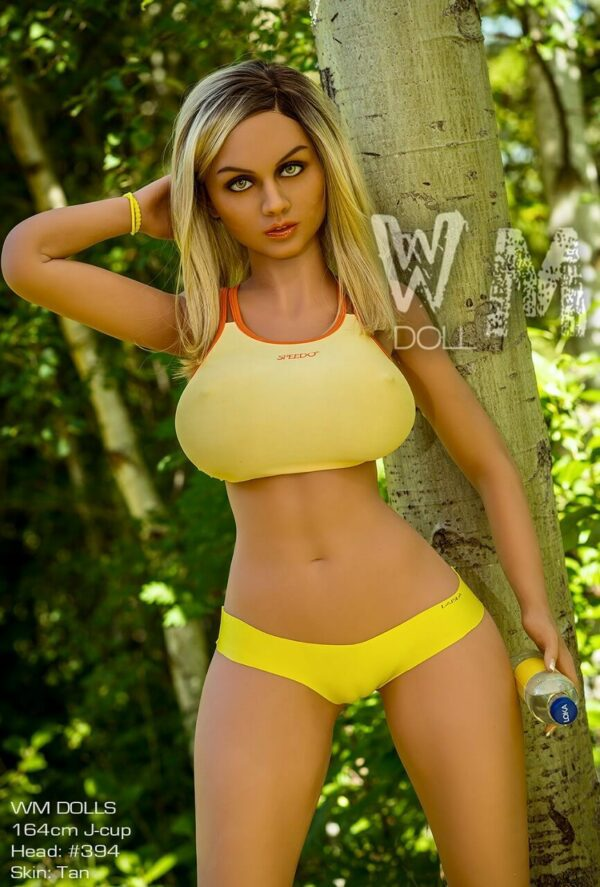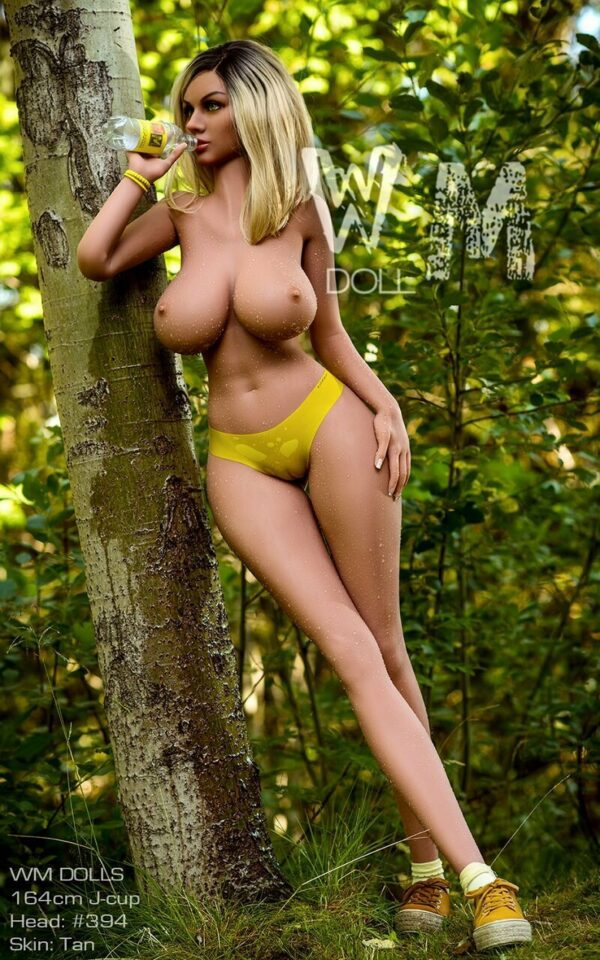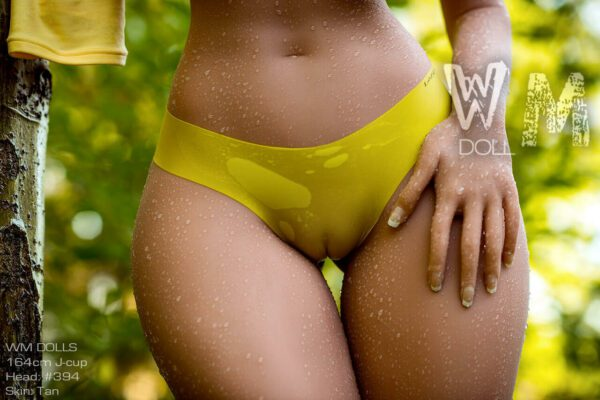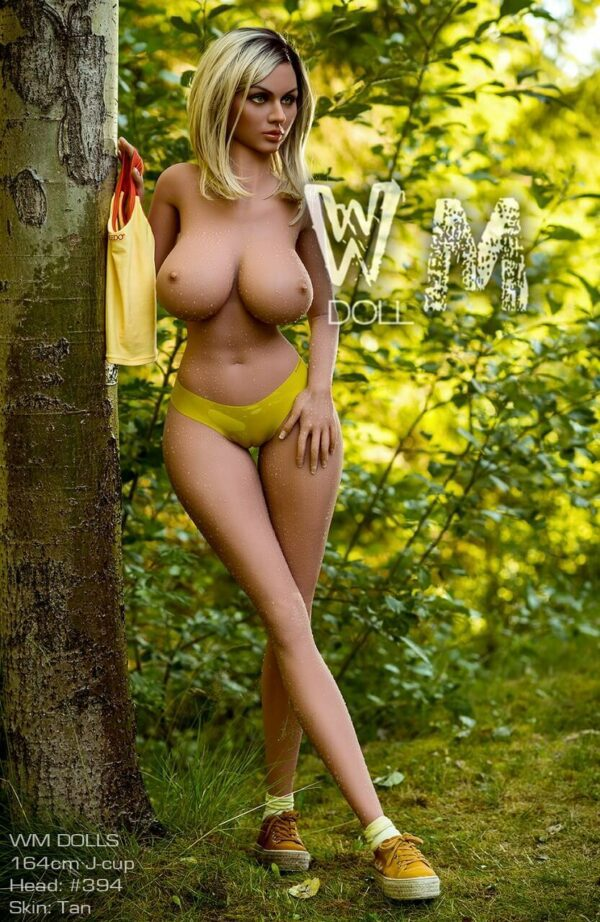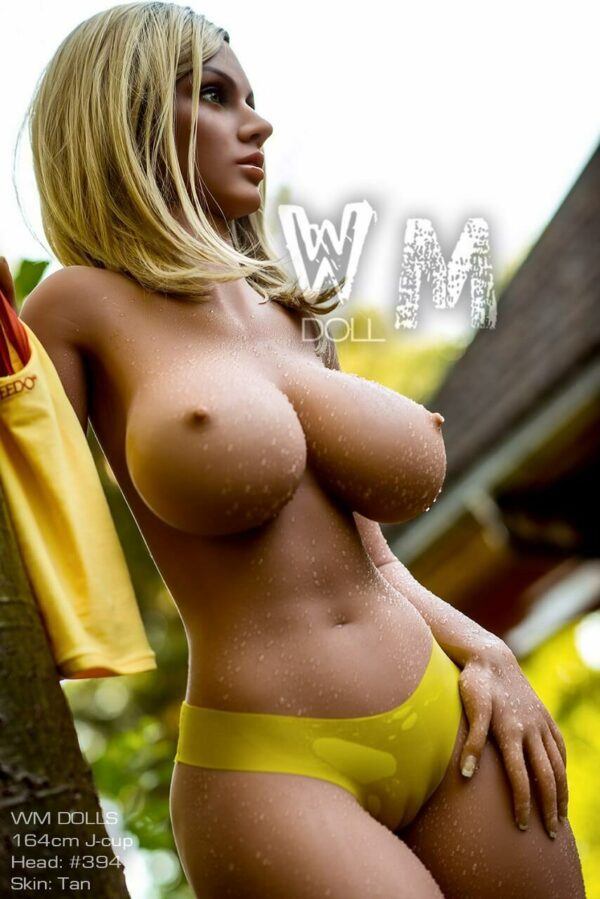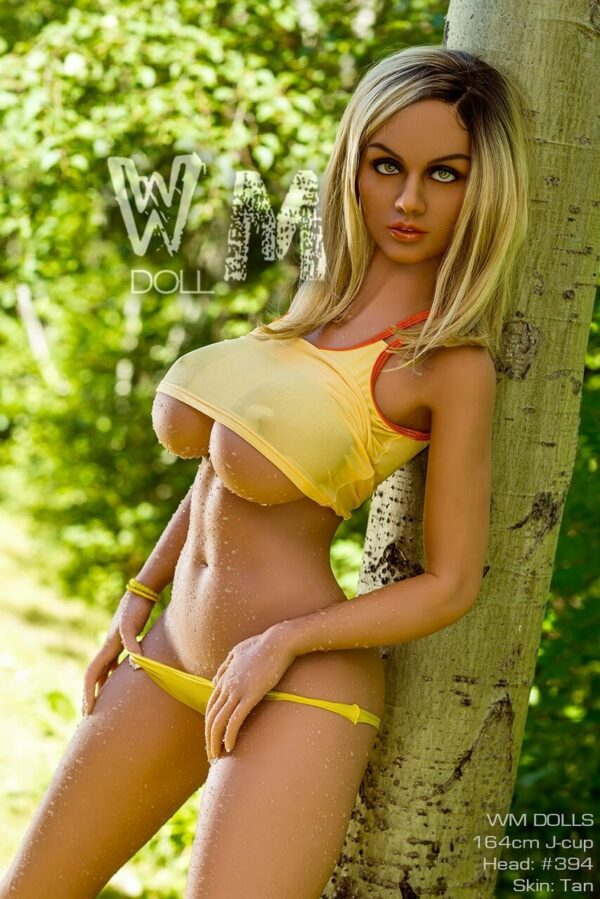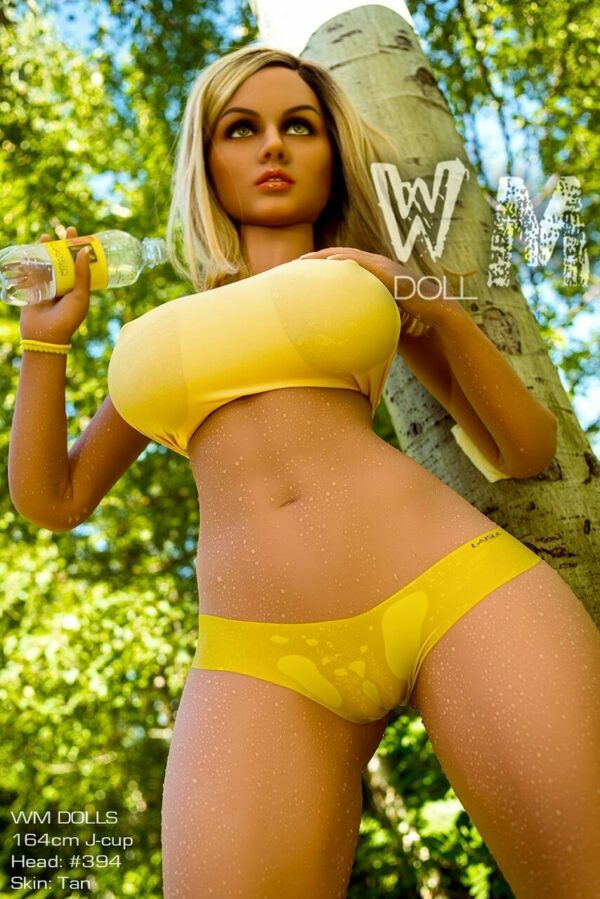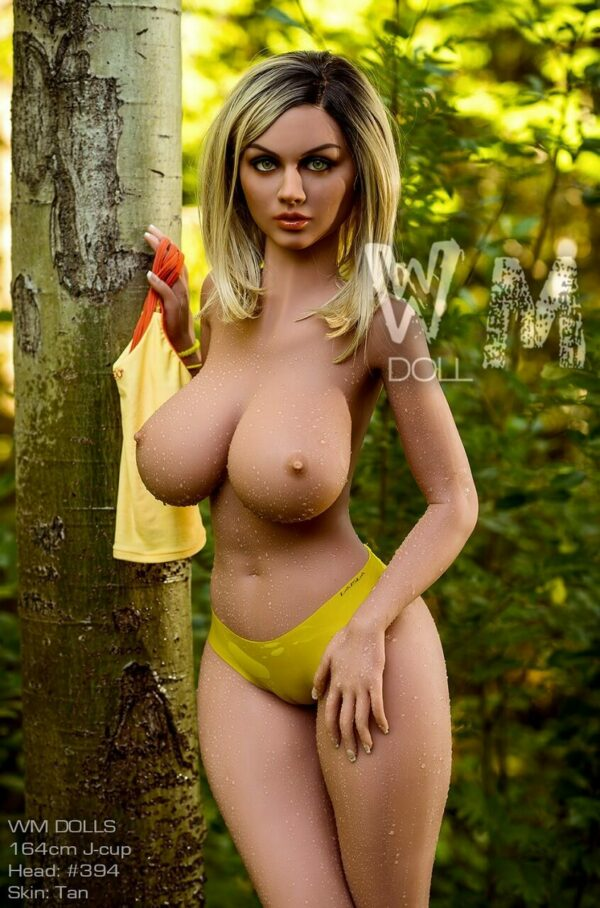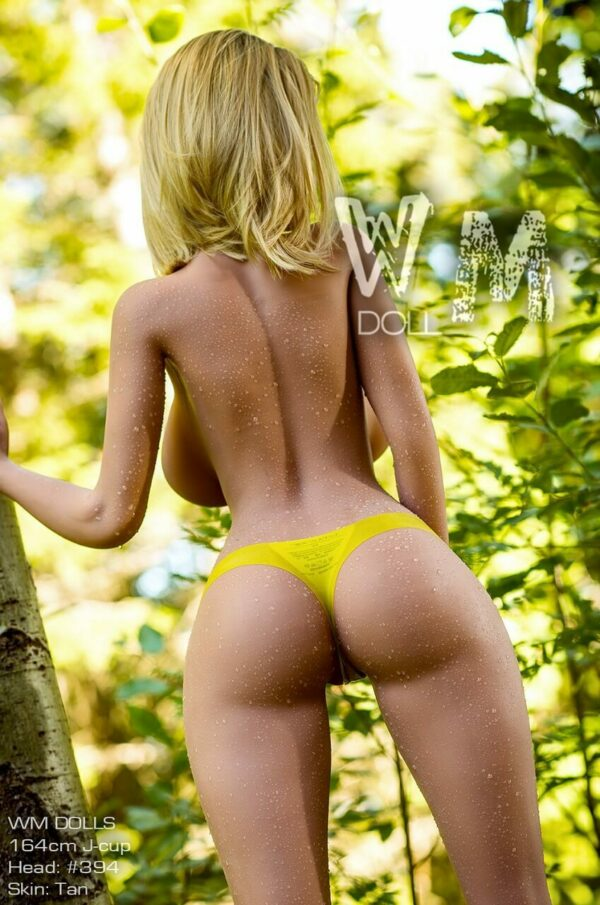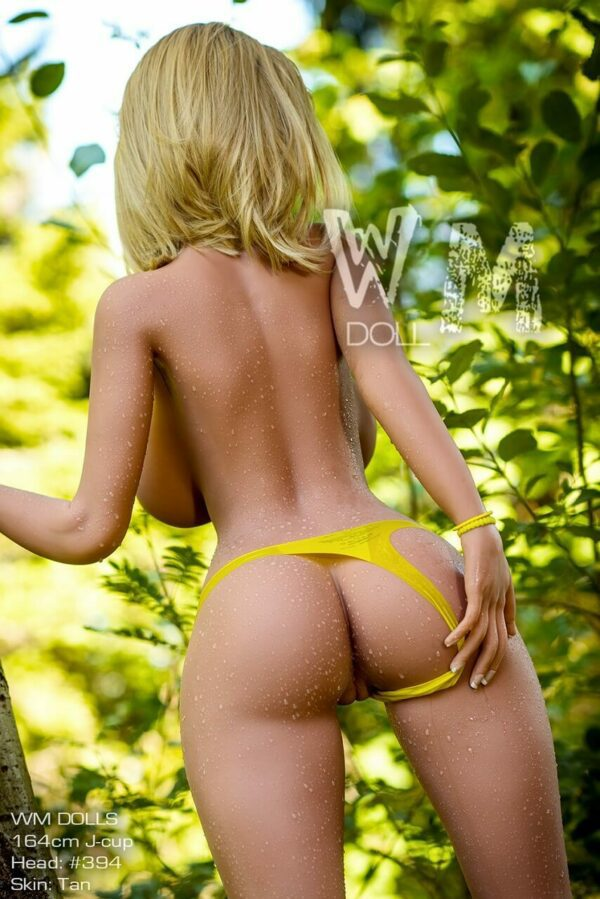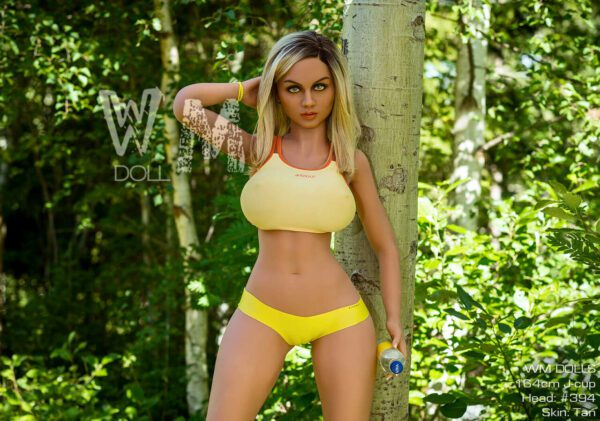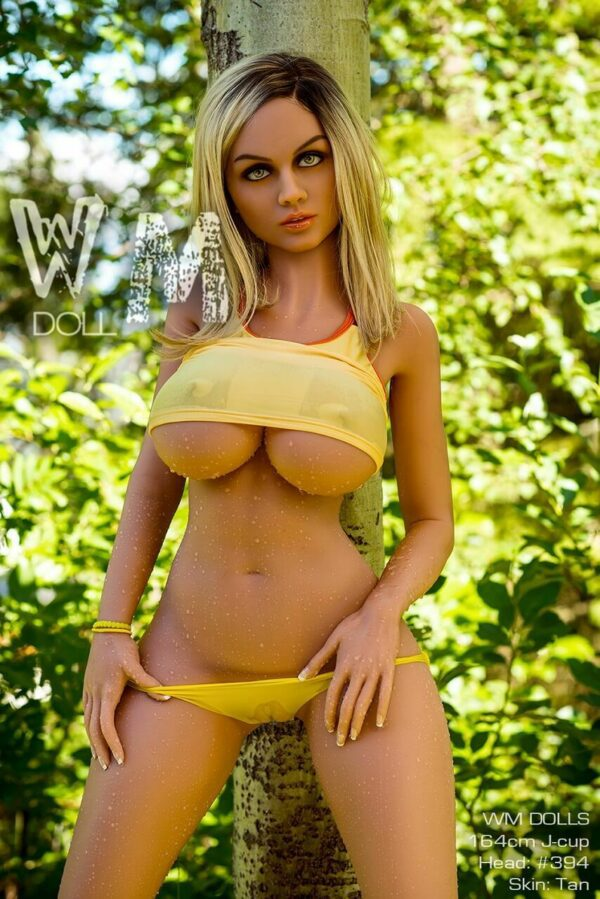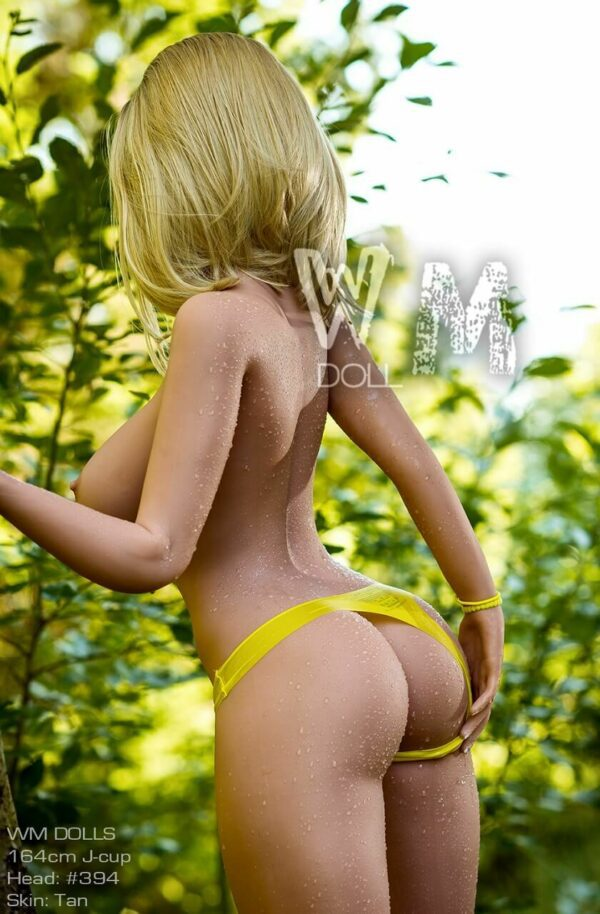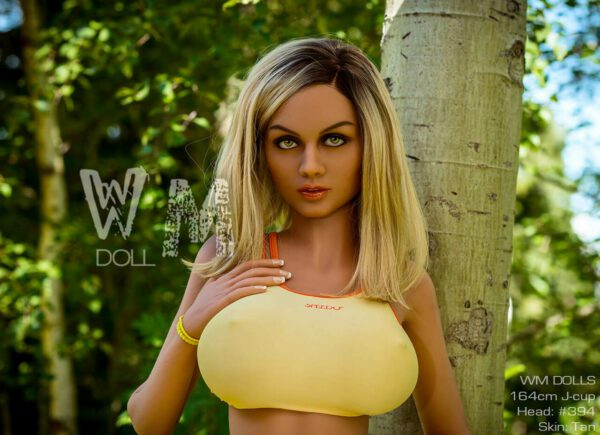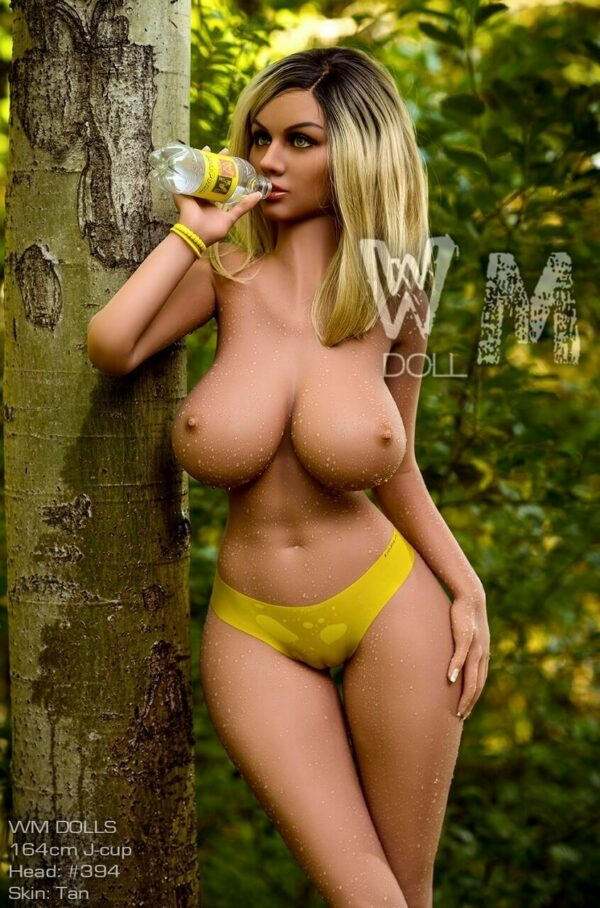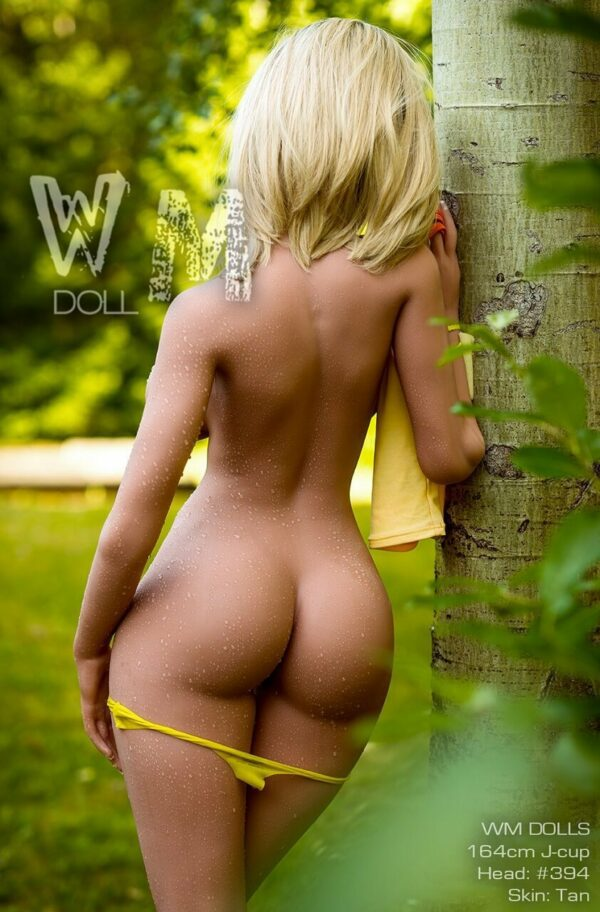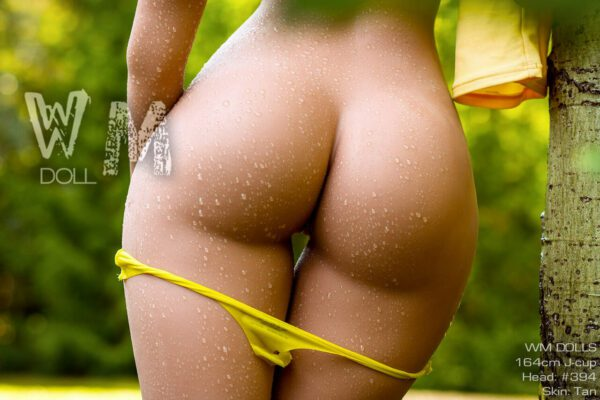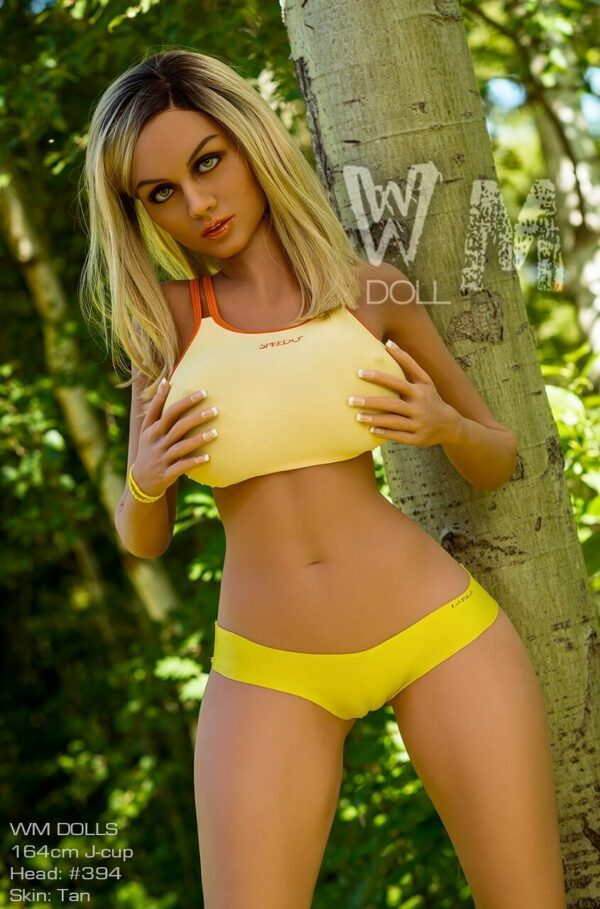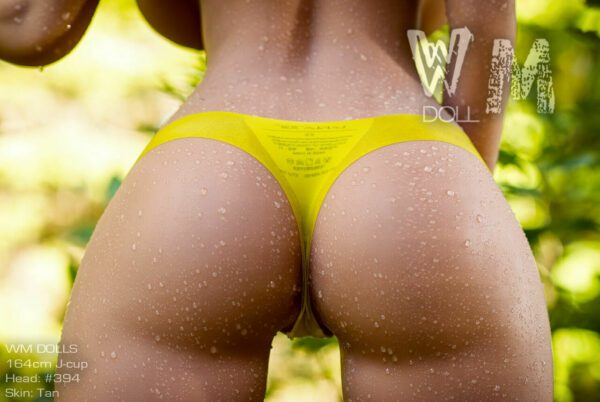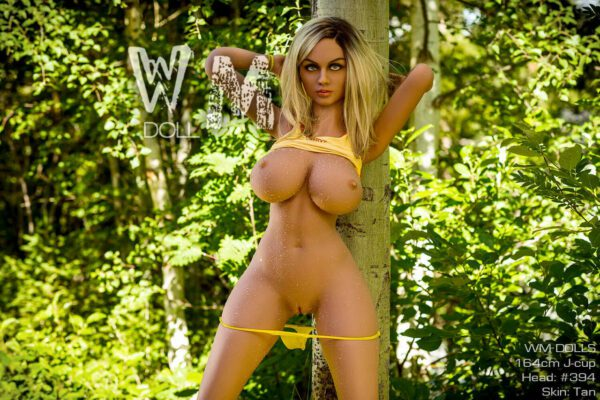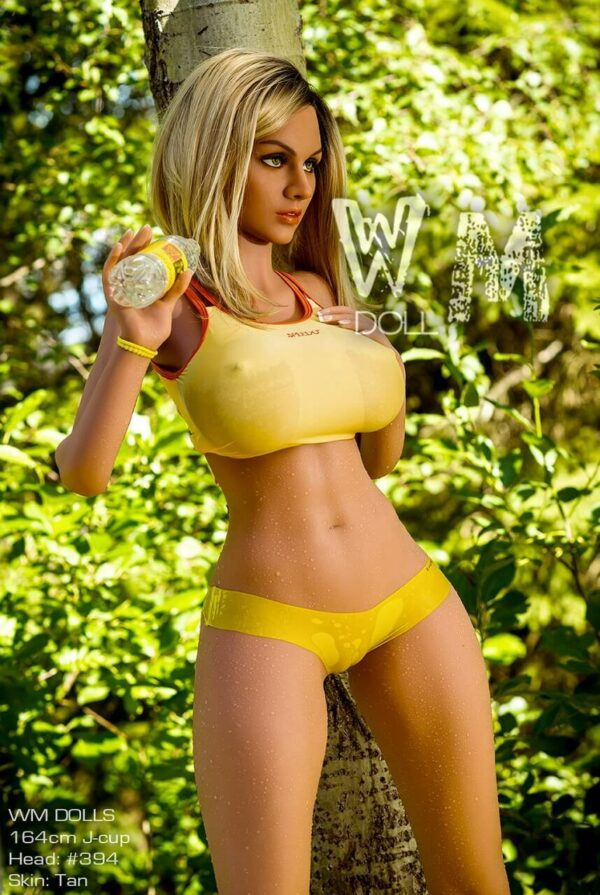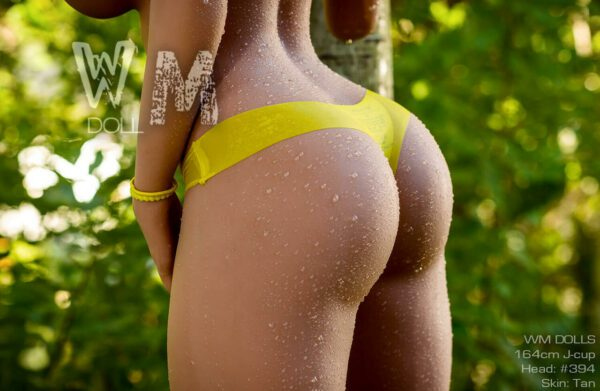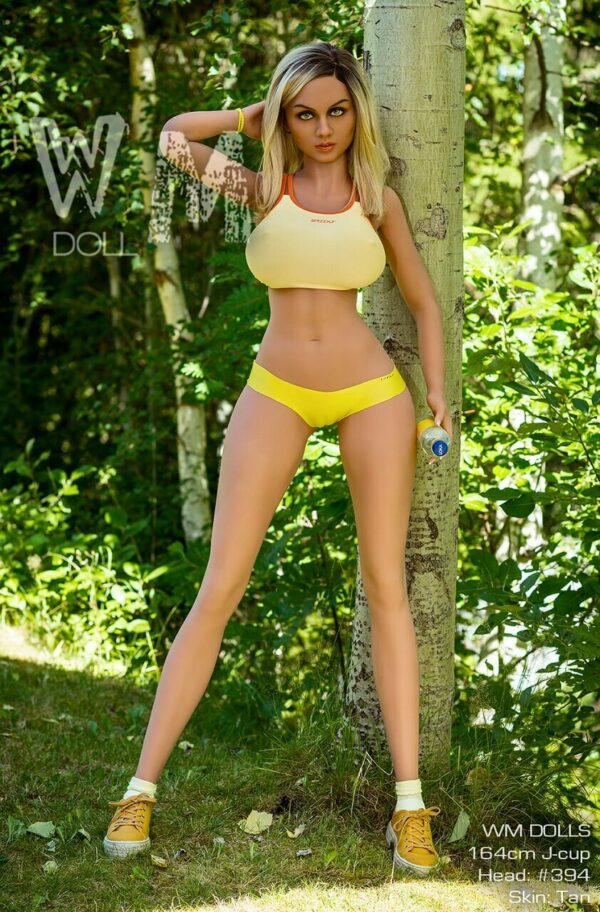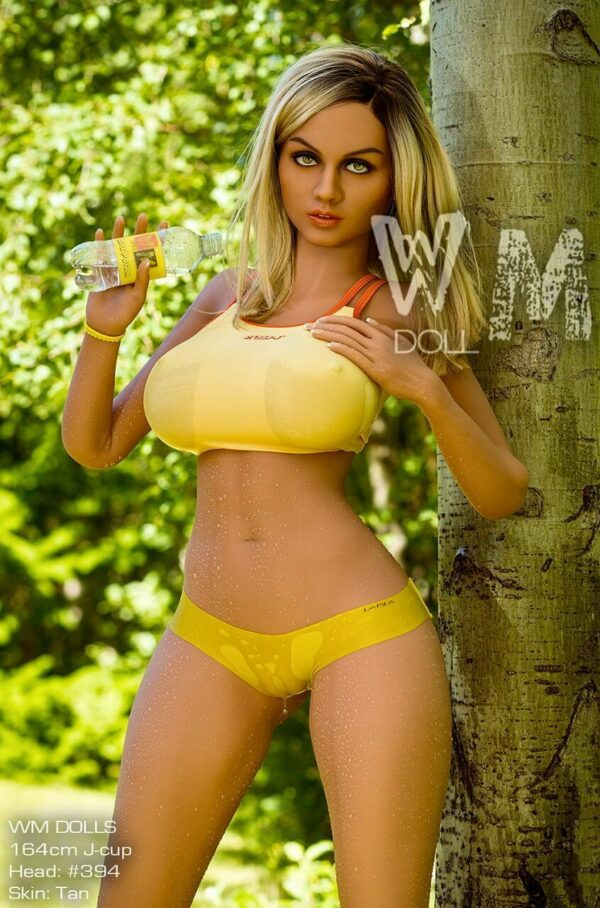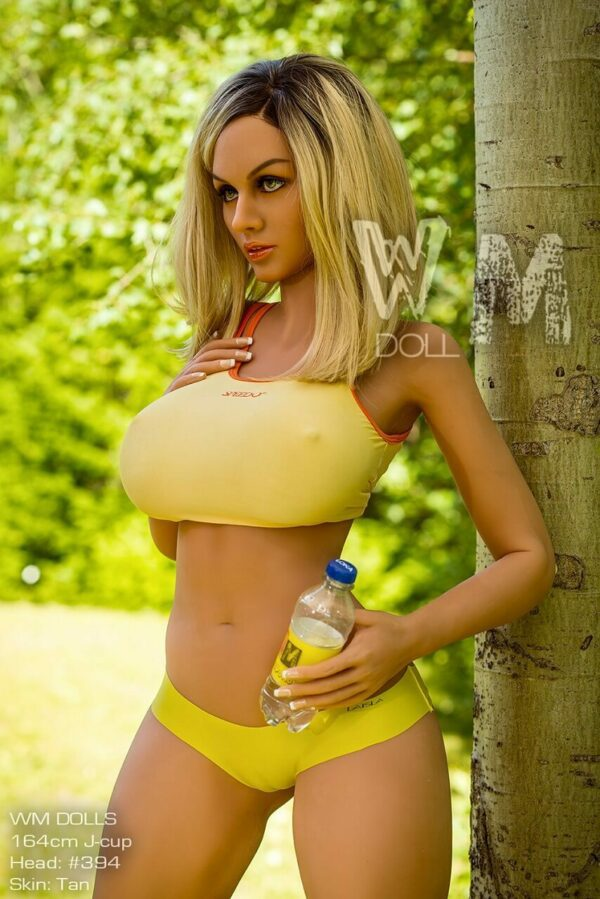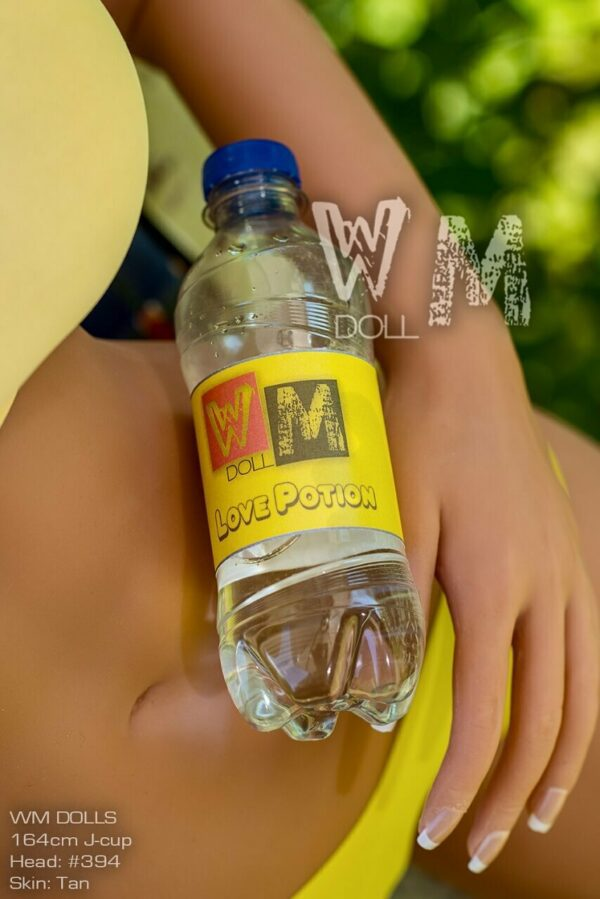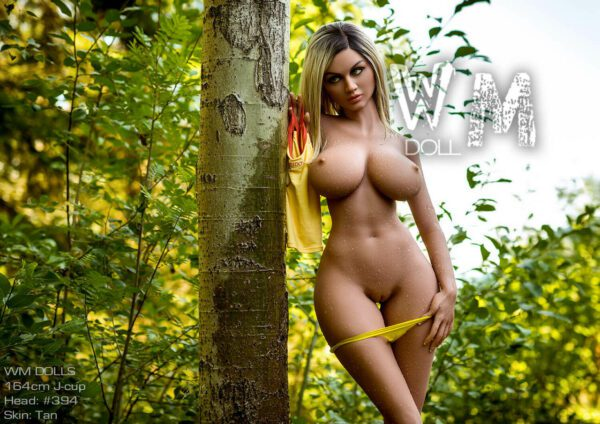# Angika – 5’5″ | 164cm Hot Sex Doll

WM Doll
\$1,700

Angika – 5’5″ | 164cm Hot Sex Doll made with high quality and safety standards by WM Doll , this genuine silicone and TPE sexy real sex doll is CE certified. With various features complementing each other, this realistic sex doll is sure to please the owner.

## Description

Height > 5ft 3.5 in – 5ft 5in (161cm – 165cm), Ethnicity > American, Ethnicity > Asian, Best Sellers, Hair Color > Blonde, Hair Color > Brunette, Body Style > Curvy, Ethnicity > European, Material > Fully TPE, Ethnicity > Interracial, Breast Size > Large, See All Sex Dolls, BRANDS > WM Doll, Age > Young Adult

• \$
• \$
• \$
• \$
• \$
• \$
• \$
• \$
• \$
• \$
• \$
• \$
• \$
• \$
• \$
• \$
• \$
• \$
• \$
• \$
• \$
• \$
• \$
• \$
• \$
• \$
• \$
• \$
• \$
• \$
• \$
• \$
• \$
• \$
• \$
• \$
• \$
• \$
• \$
• \$
• \$
• \$
• \$
• \$
• \$
• \$
• \$
• \$
• \$
• \$
• \$
• \$
• \$
• \$
• \$
• \$
• \$
• \$
• \$
• \$
• \$
• \$
• \$
• \$
• \$
• \$
• \$
• \$
• \$
• \$
• \$
• \$
• \$
• \$
• \$
• \$
• \$
• \$
• \$
• \$
• \$
• \$
• \$
• \$
• \$
• \$
• \$
• \$
• \$
• \$
• \$
• \$
• \$
• \$
• \$
• \$
• \$
• \$
• \$
• \$
• \$
• \$
• \$
• \$
• \$
• \$
• \$
• \$
• \$
• \$
• \$
• \$
• \$
• \$
• \$
• \$
• \$
• \$
• \$
• \$
• \$
• \$
• \$
• \$
• \$
• \$
• \$
• \$
• \$
• \$
• \$
• \$
• \$
• \$
• \$
• \$
• \$

• \$
• \$
• \$
• \$
• \$
• \$
• \$

• \$

• \$
• \$
• \$
• \$
• \$
• \$
• \$
• \$
• \$
• \$
• \$
• \$
• \$
• \$
• \$
• \$
• \$
• \$
• \$
• \$
• \$

• \$
• \$
• \$
• \$
• \$
• \$
• \$
• \$
• \$
• \$
• \$
• \$
• \$
• \$
• \$
• \$
• \$
• \$
• \$
• \$

• \$
• \$
• \$
• \$
• \$
• \$
• \$

• \$
• 40 \$

• \$
• 100 \$

• \$
• \$
• \$
• \$
• \$

• \$
• \$
• \$
• \$
• \$

• \$
• \$
• \$

• \$
• 130 \$

• \$
• \$
• \$

• \$
• 40 \$

• \$
• \$
• \$
• \$

• \$
• \$
• \$
• \$
• \$

• \$
• \$

• \$
• \$
• \$
• \$
• \$
• \$
• \$
• \$
• \$
• \$

• \$
• \$
• \$
• \$
• \$
• \$

• \$
• 200 \$

• \$
• 100 \$

• \$
• 675 \$

• \$
• 390 \$

## Doll Measurements

Height: 164 cm | 5 ft 4.5 in
Weight: 34kg | 74.9 lbs.
Shoulder: 34 cm | 12.2 in
Full Bust: 86 cm | 33.8 in
Under Bust: 56 cm | 22.4 in
Cup Size: J
Waist: 61 cm | 24 in
Hips: 91 cm | 35.8 in
Foot Length: 21 cm | 8.3 in
Vaginal Depth: 18cm | 7 in
Anal Depth: 15 cm | 5.9 in
Oral Depth: 12 cm | 4.7 cm